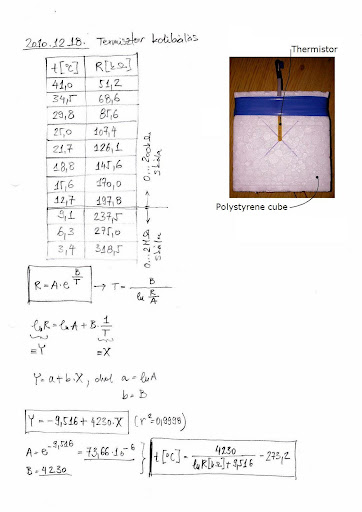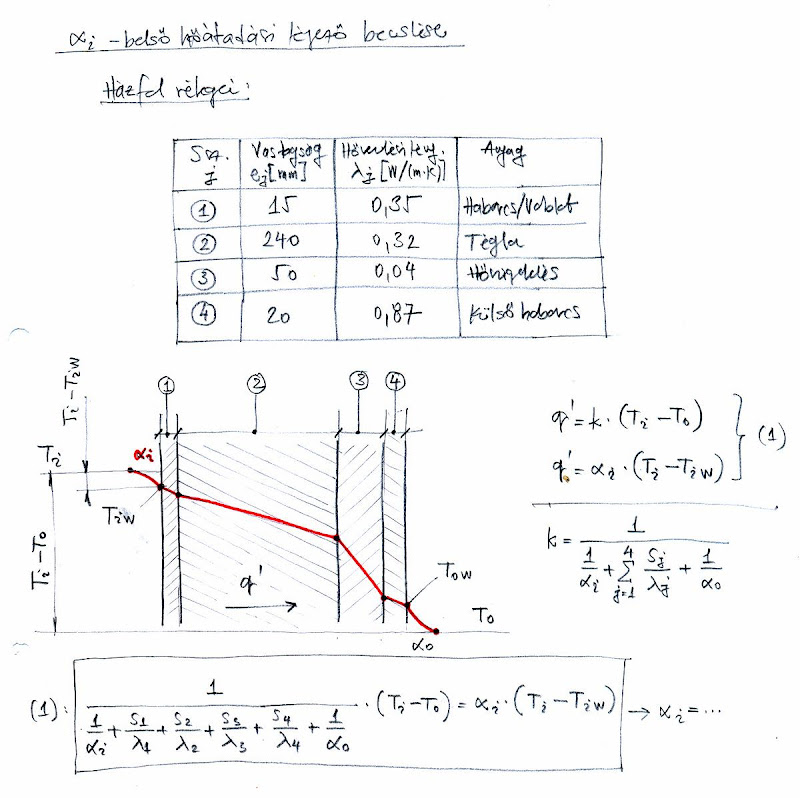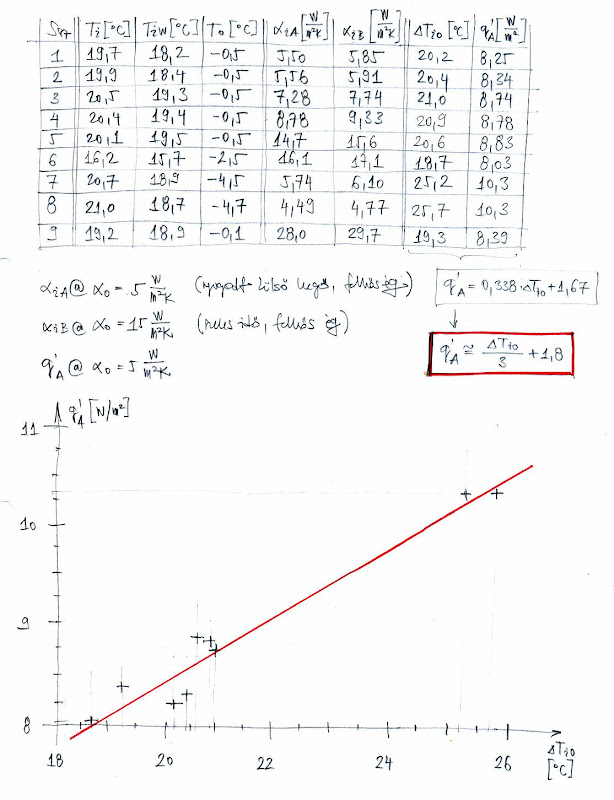The Museum of HP Calculators

HP Forum Archive 20

 Re: The lost formulaMessage #1 Posted by Csaba Tizedes (Hungary) on 20 Jan 2011, 4:40 a.m. In last December I used an equation and works very well. I want to made a house-wall-temperature-meter to calculate heat loss of my house. This was a very simple polystirene cube (bad thermal conductivity for more precise measure) and a little PSION Revo thermistor. This "device" works very simple: Push the thermistor to the wall, wait for 15-20 seconds, measure the resistance then calculate temperature. A pic about it and a calibration calculation:Re: The lost formulaMessage #2 Posted by designnut on 20 Jan 2011, 10:41 p.m.,in response to message #1 by Csaba Tizedes (Hungary) That seems precise. What method of regression analysis did you use? What calculator?,Sam

 Re: The lost formulaMessage #3 Posted by John B. Smitherman on 22 Jan 2011, 6:15 p.m.,in response to message #2 by designnut Hi Sam. I used a 35s to work through Csaba's example and of course it only does linear regression. I had to remember to take the ln of resistance and 1/Temp in degrees K before entering them as y and x. Regards, John

 Re: The lost formulaMessage #4 Posted by Csaba Tizedes (Hungary) on 26 Jan 2011, 5:25 a.m.,in response to message #2 by designnut Hi Sam, I usually use Excel to fit functions, because it's simple and compatible with everything. Sometimes I grab my old 48SX and feed them with data. This regression a simple least-squares method. The temperature need to calculate from Celsius to Kelvins!

 Re: The lost formulaMessage #5 Posted by John B. Smitherman on 20 Jan 2011, 10:55 p.m.,in response to message #1 by Csaba Tizedes (Hungary) Good work Csaba and thanks for sharing. Where did you get the temperature data used to develop the correlation? By the way, what did you learn about your house's heat loss? Regards, John

 Re: The lost formulaMessage #6 Posted by Csaba Tizedes (Hungary) on 26 Jan 2011, 5:59 a.m.,in response to message #5 by John B. Smitherman I have a good mercury-filled thermometer (scale divided into 0.5C from 0C-100C, and approximate the temperature +-0.1C). I used a simple plasticware, filled with ~half liter water then I was cooled slowly with snow. If temperature not changed I was read a measuring point data. Some approximately data about my house wall's heat loss: 1.) I assume that the wall have four layer (see the table: 2nd is the brick layer, 3rd is the heat-isolation layer and 1st and 4th the inner and outer mortar/grout (I dont know how to say it in English)). 2.) I want to know the inner heat transfer coefficient (alpha_i [W/(m2*K)]), so I wrote a heat flux from dining-room wall "inner" side to "outer" (ambient) , then I wrote some equation from "inner" side to inner wall surface. In this points the temperatures measurable (Ti, Tiw, To), and then I solved this equation (1) to alpha_i. 3.) With this coefficient the heat flux calculated: q'A [W/m2]=alpha_i*(Ti-Tiw) 4.) Then I plot this heat flux as function of temperature difference. Unfortunately I have not measuring points yet at dT=22...24C. Scans about it:The coloumn alpha_i_A calculated at alpha_o=5 W/(m2*K) (no wind, cloudy weather) and alpha_i_B calculated at alpha_o=15 W/(m2*K) (windy, cloudy weather)Go back to the main exhibit hall